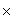From: Chris I have a sum of cash that I want to use as a deposit \$ 5000 and a lender will lend me 65% of the purchase price. If the purchase price is is \$ 10 000 then 65% is 6500 so I would need 10 000 - 6500 = 3500. How do I calclate the maximum value I can borrow if I konw I have \$5000 cash and the lender will lend 65% ? Hi Chris. If the lender will give you 65% of the price then you have to provide 35% of the price. You have \$ 5 000, so that is your 35%. You need to figure out what total price this is. Let's call the total T: 35%T = \$ 5 000 Now you can re-arrange this to solve for T, your maximum purchase price. To figure out how much of this you'd be borrowing, just subtract the \$5 000 you are putting up as the deposit. Hope this helps! Sue